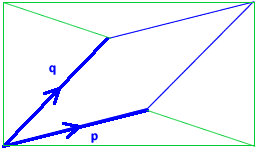#### You may also like### At a Glance

The area of a regular pentagon looks about twice as a big as the pentangle star drawn within it. Is it?### Six Discs

Six circular discs are packed in different-shaped boxes so that the discs touch their neighbours and the sides of the box. Can you put the boxes in order according to the areas of their bases?### Equilateral Areas

ABC and DEF are equilateral triangles of side 3 and 4 respectively. Construct an equilateral triangle whose area is the sum of the area of ABC and DEF.

# Areas of Parallelograms

##### Age 14 to 16 Challenge Level:

Tony sent us his answers to the first parts.

(a) $6$ units squared ($=3\times 2-0\times 5$)
(b) $12$ units squared ($=3\times 4-2\times 0$)
(c) $9$ units squared ($=4\times 3-1\times 3$)
(d)$10$ units squared ($=2\times 3-4\times -1$)

James, Jamie and George from Gillingham School sent us their formula for the area of the general parallelogram.

$$a d-b c\ \text{ (or } bc-ad\text{ if } bc>ad)$$

Here's one way to work that out.Remember that $\mathbf{p}=\left(\begin{array}{c}a \\ b\end{array}\right)$ and $\mathbf{q}=\left(\begin{array}{c}c\\d\end{array}\right)$.

The diagram shows the parallelogram inside a rectangle with base $a+c$ and height $b+d$. This rectangle has area $(a+c)(b+d)$. If we could find the areas of the triangles outside the parallelogram, then we could subtract them from the area of the rectangle to find the area of the parallelogram.

The bottom triangle has height $b$ and base $a+c$, so has area $\frac{1}{2}b(a+c)$.

The triangle on the left has (if you imagine it on its side so that its base is at the bottom) base $b+d$ and height $c$, so has area $\frac{1}{2}c(b+d)$.

The triangle at the top is congruent to the triangle at the bottom (it has been rotated by $180^{\circ}$), so has area $\frac{1}{2}b(a+c)$.

The triangle on the right is congruent to the triangle on the left, so has area $\frac{1}{2}c(b+d)$.

So the area of the parallelogram is $$(a+c)(b+d)-2\times\frac{1}{2}b(a+c) -2\times\frac{1}{2}c(b+d)=$$
$$a b+b c+a d+c d-a b-b c-b c-c d=a d-b c$$

just as James, Jamie and George said.